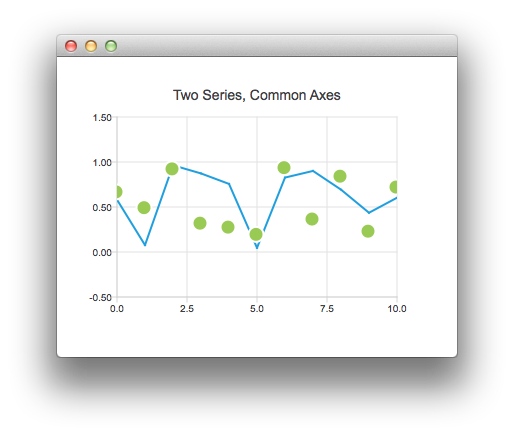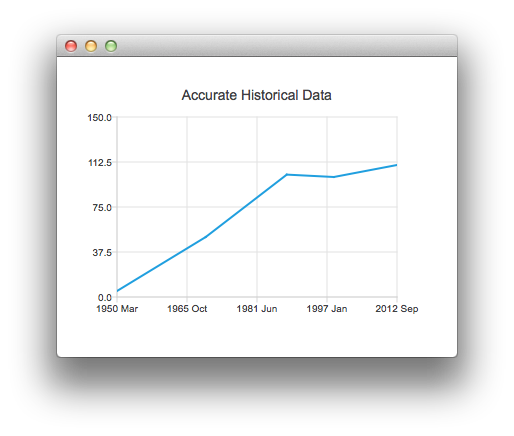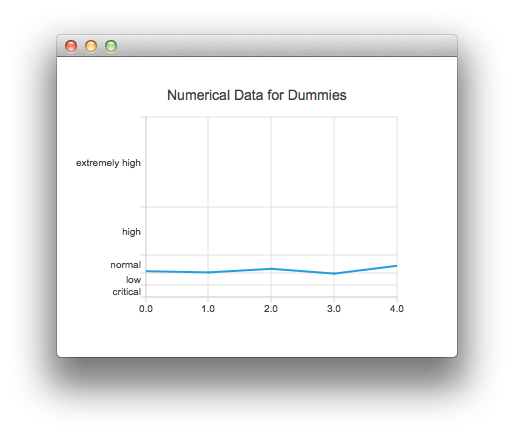# Qml Axes

This is a demonstration of how to use axes in your QML application.

#### Running the Example

To run the example from Qt Creator, open the Welcome mode and select the example from Examples. For more information, visit Building and Running an Example.

#### Using Axes in Qt Quick Applications

We begin with a chart that has a line series and a scatter series with random data. Both series use the same axes.```ChartView {
title: "Two Series, Common Axes"
anchors.fill: parent
legend.visible: false
antialiasing: true

ValueAxis {
id: axisX
min: 0
max: 10
tickCount: 5
}

ValueAxis {
id: axisY
min: -0.5
max: 1.5
}

LineSeries {
id: series1
axisX: axisX
axisY: axisY
}

ScatterSeries {
id: series2
axisX: axisX
axisY: axisY
}
}

// Add data dynamically to the series
Component.onCompleted: {
for (var i = 0; i <= 10; i++) {
series1.append(i, Math.random());
series2.append(i, Math.random());
}
}```

The next example shows a chart with some accurate historical data that makes us to use a DateTimeAxis.```ChartView {
title: "Accurate Historical Data"
anchors.fill: parent
legend.visible: false
antialiasing: true

LineSeries {
axisX: DateTimeAxis {
format: "yyyy MMM"
tickCount: 5
}
axisY: ValueAxis {
min: 0
max: 150
}

// Please note that month in JavaScript months are zero based, so 2 means March
XYPoint { x: toMsecsSinceEpoch(new Date(1950, 2, 15)); y: 5 }
XYPoint { x: toMsecsSinceEpoch(new Date(1970, 0, 1)); y: 50 }
XYPoint { x: toMsecsSinceEpoch(new Date(1987, 12, 31)); y: 102 }
XYPoint { x: toMsecsSinceEpoch(new Date(1998, 7, 1)); y: 100 }
XYPoint { x: toMsecsSinceEpoch(new Date(2012, 8, 2)); y: 110 }
}
}

// DateTimeAxis is based on QDateTimes so we must convert our JavaScript dates to
// milliseconds since epoch to make them match the DateTimeAxis values
function toMsecsSinceEpoch(date) {
var msecs = date.getTime();
return msecs;
}```

And the final example with a chart that uses a CategoryAxis to make the data easier to understand.```ChartView {
title: "Numerical Data for Dummies"
anchors.fill: parent
legend.visible: false
antialiasing: true

LineSeries {
axisY: CategoryAxis {
min: 0
max: 30
CategoryRange {
label: "critical"
endValue: 2
}
CategoryRange {
label: "low"
endValue: 4
}
CategoryRange {
label: "normal"
endValue: 7
}
CategoryRange {
label: "high"
endValue: 15
}
CategoryRange {
label: "extremely high"
endValue: 30
}
}

XYPoint { x: 0; y: 4.3 }
XYPoint { x: 1; y: 4.1 }
XYPoint { x: 2; y: 4.7 }
XYPoint { x: 3; y: 3.9 }
XYPoint { x: 4; y: 5.2 }
}
}```

Example project @ code.qt.io